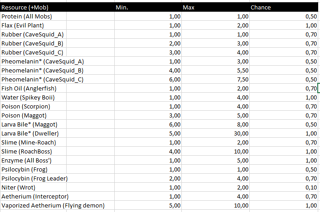A screenshot posted on the Mechanic Miner Discord by HappyNeedo showing the core drop chance (min. amount / max. amount / drop chance) of all the drop-able resources in the game.

Drop chance refers to the chance and amount of a specific resource dropping when harvesting a corpse of a dead mob.

## Core drop chance

Each mob has a set of resources they can drop. When the corpse of the dead mob is harvested, each possible resource will separately roll whether to drop or not depending on a set drop chance. If the roll is successful, the mob will drop between a core minimum and core maximum amount of that specific resource.

Example:
The Scorpion has a 0.7 (70%) chance of dropping between 1 and 4 Venom. If the drop chance is successfully rolled, there's an equal 25% chance of the Scorpion dropping either 1, 2, 3 or 4 Venom.

## Depth modifier

The drop chance is always modified by a depth modifier which modifies the core min. and max. amount of resources dropped depending on the depth level in which the mob was killed. Each depth level multiplies the min. amount by 0.25 (25%) and the max. amount by 0.5 (50%) - the actual chance of the resource dropping is non affected.

Example:
The Bat can (like any mob) drop Protein which has a core min. amount of 1 and core max. amount of 1.

If a Bat is killed and harvested at depth level 1 it will drop:

```Min. amount = core min. amount * (1 + (Depth level * min. amount multiplier) = 1 * (1 + (1 * 0.25) = 1.25 (rounded to 1)
Max. amount = core max. amount * (1 + (Depth level * max. amount multiplier) = 1 * (1 + (1 * 0.50) = 1.50 (rounded to 2)
A Bat at depth level 1 can drop between 1 and 2 Protein at a 50% chance.
```

On the other hand, if a Bat is killed and harvested at depth level 13 it will drop:

```Min. amount = core min. amount * (1 + (Depth level * min. amount multiplier) = 1 * (1 + (13 * 0.25) = 4.25 (rounded to 4)
Max. amount = core max. amount * (1 + (Depth level * max. amount multiplier) = 1 * (1 + (13 * 0.50) = 7.50 (rounded to 8)
A Bat at depth level 13 can drop between 4 and 8 Protein at a 50% chance.
```

Thus, reaching a higher depth level will yield more loot from mobs. The highest depth level is currently 15 which is the lowest level in the Deep Cave.